# PSAT Math : How to find the solution to an inequality with multiplication

## Example Questions

### Example Question #31 : Inequalities

If –1 < n < 1, all of the following could be true EXCEPT:

(n-1)2 > n

n2 < n

|n2 - 1| > 1

16n2 - 1 = 0

n2 < 2n

|n2 - 1| > 1

Explanation: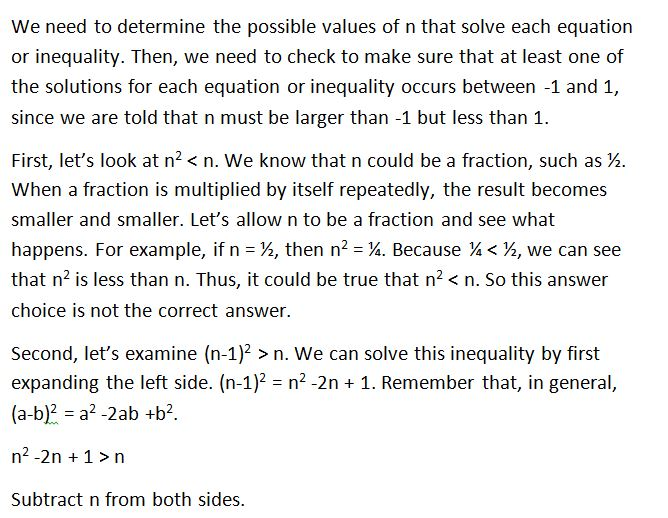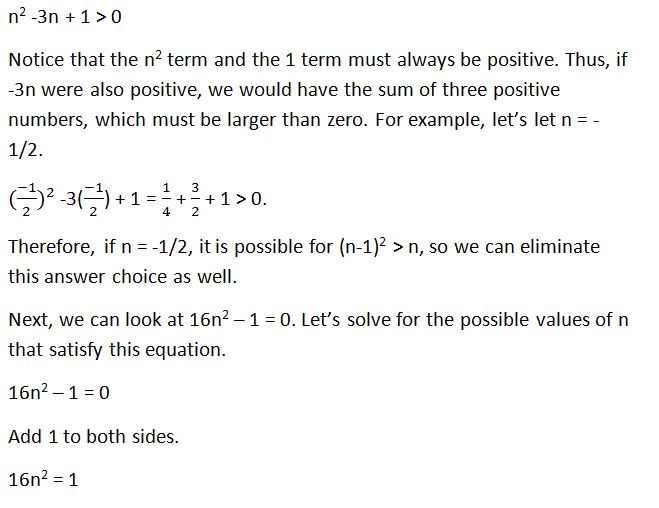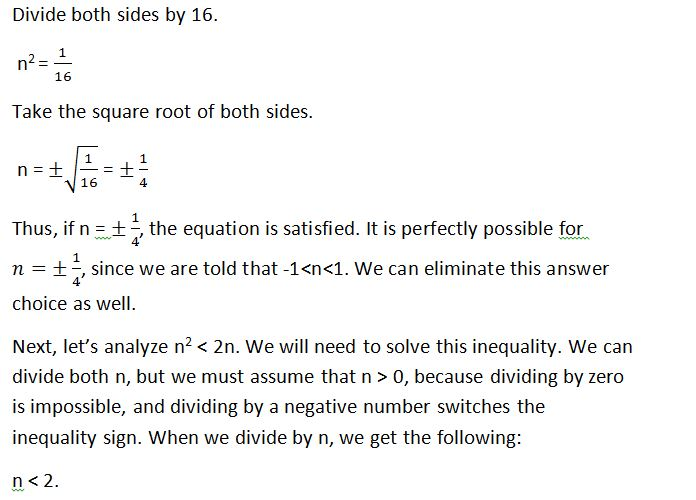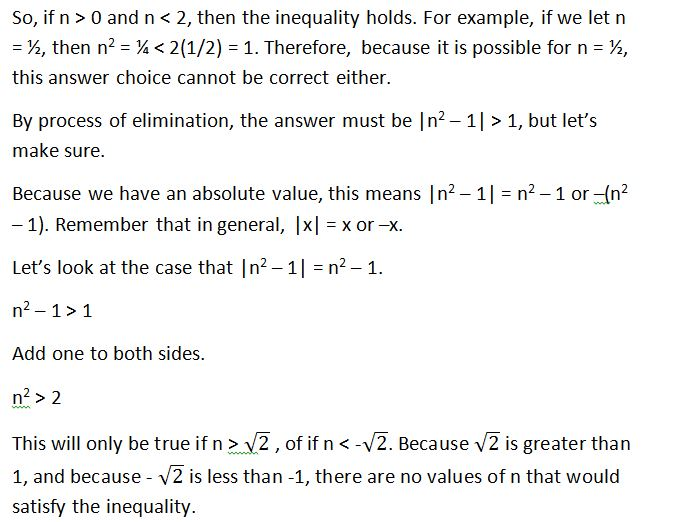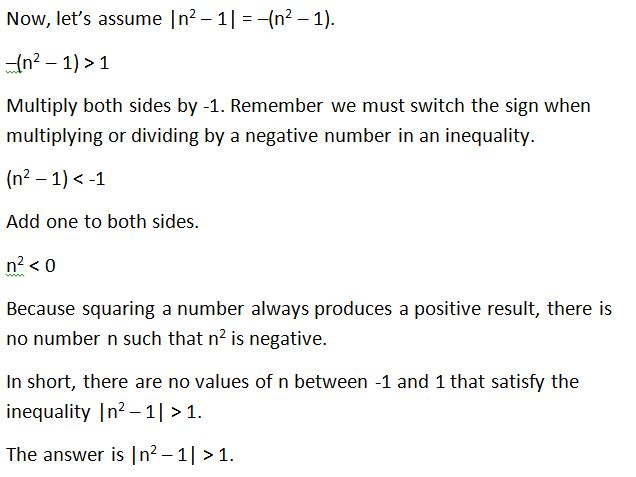### Example Question #32 : Inequalities

(√(8) / -x ) <  2. Which of the following values could be x?

All of the answers choices are valid.

-3

-1

-2

-4

-1

Explanation:

The equation simplifies to x > -1.41. -1 is the answer.

### Example Question #1 : How To Find The Solution To An Inequality With Multiplication

Solve for x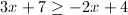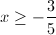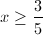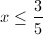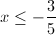Explanation: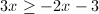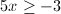### Example Question #34 : Inequalities

We have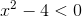, find the solution set for this inequality.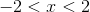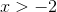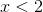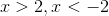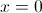Explanation: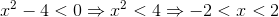### Example Question #32 : Inequalities

Fill in the circle with either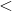,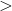, orsymbols: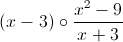for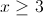.

The rational expression is undefined.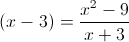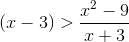None of the other answers are correct.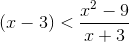Explanation:Let us simplify the second expression. We know that: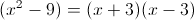So we can cancel out as follows: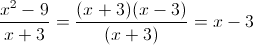### Example Question #21 : Inequalities

What is the greatest value ofthat makes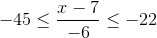a true statement?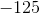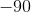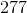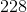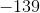Explanation:

Find the solution set of the three-part inequality as follows: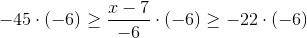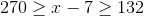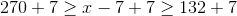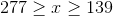The greatest possible value ofis the upper bound of the solution set, which is 277.

### Example Question #22 : Inequalities

What is the least value ofthat makesa true statement?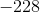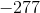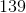Explanation:

Find the solution set of the three-part inequality as follows:The least possible value ofis the lower bound of the solution set, which is 139.

### Example Question #23 : Inequalities

Give the solution set of the inequality: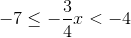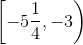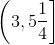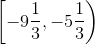None of the other responses gives the correct answer.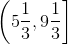Explanation:

Divide each of the three expressions by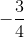, or, equivalently, multiply each by its reciprocal,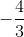: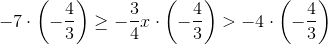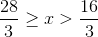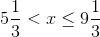or, in interval form,.

### Example Question #24 : Inequalities

Give the solution set of the following inequality: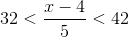None of the other responses gives the correct answer.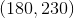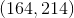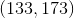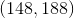Explanation: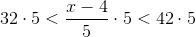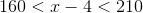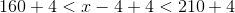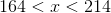or, in interval notation,.

### Example Question #25 : Inequalities

Which of the following numbers could be a solution to the inequality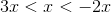?